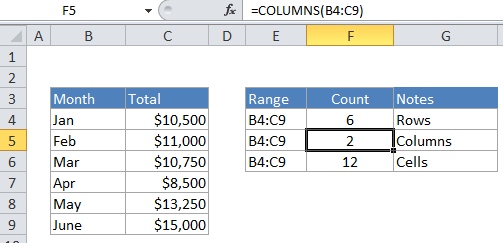## Excel Office

Excel How Tos, Tutorials, Tips & Tricks, Shortcuts

# How to count total columns in range in Excel

If you need to count the number of columns in a range, use the COLUMNS function.
COLUMNS counts the number of columns in and returns the result as a number.

## Formula

`=COLUMNS(range)`Worked Example:   How to get relative row numbers in a range in Excel

## Explanation

In the example, the active cell contains this formula:

`=COLUMNS(B4:C9)`